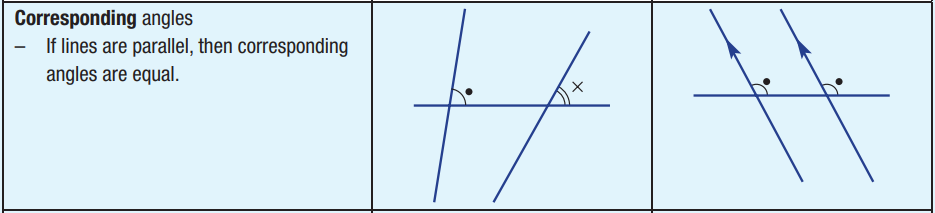# Corresponding Angles

Topic:
Angles
When two parallel lines are crossed by another line (which is called the Transversal), the angles in matching corners are called corresponding angles. When the two lines being crossed are parallel, the corresponding angles formed by the transversal are equal. Use the animations below to explore, visualize and verify corresponding angles.

## SummaryIf the two lines are not parallel, then the corresponding angles formed by the transversal are not equal. When the two lines are parallel (the 'arrow' markings tell you this), the transversal forms corresponding angles that are equal.